# RS Aggarwal Class 10 Solutions Chapter 3 - Linear Equations In Two Variables - Ex 3A(3.1)

## RS Aggarwal Class 10 Chapter 3 - Linear Equations In Two Variables - Ex 3A(3.1) Solutions Free PDF

The RS Aggarwal Class 10 solutions Chapter 3 is considered an ideal way of preparing for the Class 10 Maths examination of CBSE students. The exercise questions of the RS Aggarwal Class 10 Maths textbook gives an in-depth exposure of Class 10 mathematical problems which will help you to score well in your examination as well as very helpful in clearing competitive exams. All the solutions provided here are prepared and designed by highly skilled subject experts.

You should practice the exercise questions thoroughly without looking into the solutions unless and until you are stuck while solving any of the questions. It is prepared as per the CBSE Class 10 syllabus and covers all the topics mentioned in it. Students should practice exercise questions of RS Aggarwal Solutions Class 10 Chapter 3 – Linear Equations In Two Variables so that they can attempt the question paper with full confidence.

## Download PDF of RS Aggarwal Class 10 Solutions Chapter 3 – Linear Equations In Two Variables

Question 1: On a graph paper, draw a horizontal line X’OX and a vertical line YoY’ as the x-axis and the y-axis respectively.

2x – 5y + 4 = 0, 2x + y – 8 = 0

Solutions:

Given equations are 2x – 5y + 4 = 0 and 2x + y – 8 = 0

Graph of 2x – 5y + 4 = 0:

2x – 5y + 4 = 0 $\Rightarrow y = \frac{2x + 4}{5}$

Putting x = -2, we get y = 0

Putting x = 3, we get y = 2

Putting x = 8, we get y = 4

Hence, the table is,

x-238y024

Plot the points A(-2, 0), B(3, 2) and C(8, 4) on the graph paper. Join AB and BC to get the graph line AC. Extend it both ways.

Thus, line AC is the graph of 2x – 5y + 4 = 0.

Graph of 2x + y – 8 = 0:

2x + y – 8 = 0 $\Rightarrow y = -2x + 8$

Putting x = 1, we get y = 6

Putting x = 3, we get y = 2

Putting x = 2, we get y = 4

Hence, the table is,

x132y624

Now, on the same graph paper as above plot the points P(1, 6) and Q(2, 4). The point B(3, 2) has already been plotted. Join PQ and QB. Thus, the line PB is the graph of 2x + y – 8 = 0.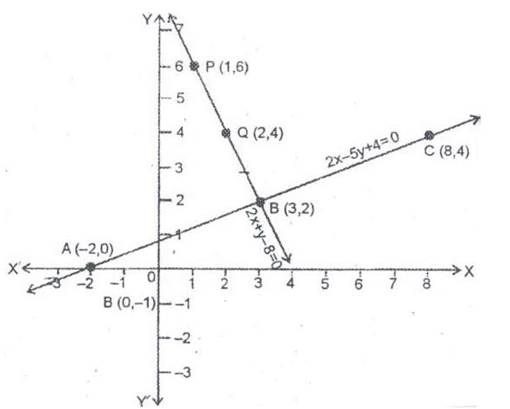The two graph lines intersect at B(3, 2). Therefore, x = 3, y = 2 is the solution of given system of equations.

Question 2: On a graph paper, draw a horizontal line X’OX and a vertical line YoY’ as the x-axis and the y-axis respectively.

3x + y + 1 = 0,

2x + y – 8 = 0

Solution:

Given equations are 3x + y + 1 = 0 and 2x + y – 8 = 0

Graph of 3x + y + 1 = 0:

3x + y + 1 = 0 $\Rightarrow y = -3 – 1$

Putting x = 0, we get y = -1

Putting x = -1, we get y = 2

Putting x = 1, we get y = -4

Hence, the table is,

x0-11y-12-4

Plot the points A(0, -1), B(-1, 2) and C(1, -4) on the graph paper. Join AB and AC to get the graph line BC. Extend it both ways.

Thus, line AC is the graph of 3x + y + 1 = 0.

Graph of 2x + y – 8 = 0:

2x + y – 8 = 0 $\Rightarrow y = \frac{-2x + 8}{3}$

Putting x = -1, we get y = 2

Putting x = 2, we get y = 4

Putting x = -4, we get y = 0

Hence, the table is,

x-12-4y240

Now, on the same graph paper as above plot the points P(2, 4) and Q(-4, 0). The point B(-1, 2) has already been plotted. Join PB and BQ. Thus, the line PQ is the graph of 2x + y – 8 = 0.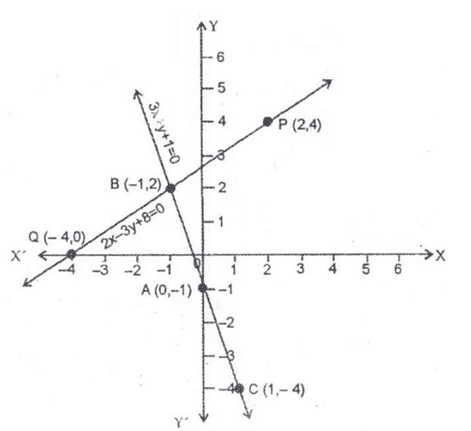The two graph lines intersect at B(-1, 2). Therefore, x = -1, y = 2 is the solution of given system of equations.

Question 3: On a graph paper, draw a horizontal line X’OX and a vertical line YoY’ as the x-axis and the y-axis respectively.

3x – 2y + 2 = 0,

(3/2) x – y + 3 = 0

Solution:

Given equations are 3x – 2y + 2 = 0 and (3/2)x – y + 3 = 0

Graph of 3x – 2y + 2 = 0:

3x – 2y + 2 = 0 $\Rightarrow y =\frac{3 + 2}{2}$

Putting x = 0, we get y = 1

Putting x = 2, we get y = 4

Putting x = 2, we get y = -2

Hence, the table is:

x02-2y14-2

Plot the points A(0, 1), B(2, 4) and C(-2, -2) on the graph paper. Join AB and AC to get the graph line BC. Extend it both ways.

Thus, line AC is the graph of 3x + y + 1 = 0.

Graph of (3/2)x – y + 3 = 0:

(3/2)x – y + 3 = 0 $\Rightarrow y = \frac{3}{2}x + 3$

Putting x = 0, we get y = 3

Putting x = 2, we get y = 6

Putting x = -2, we get y = 0

Hence, the table is,

x02-2y360

Now, on the same graph paper as above plot the points P(0, 3) and Q(2, 6) and R(-2, 0). Join PQ and PR to get QR. Thus, the line QR is the graph of (3/2)x – y + 3 = 0.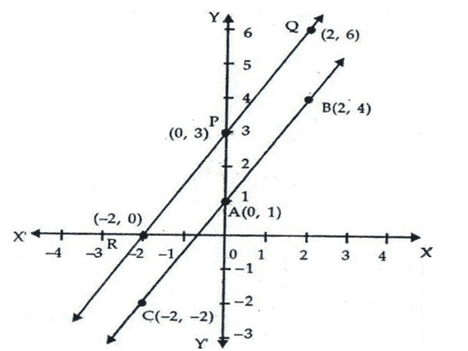It is clear from the graph that the two lines are parallel and do not intersect even when produced.

Therefore, given equations are inconsistent has no solution.

The coordinates of the points where these, lines meet y-axis are A(0, 1) and B(0, 3) respectively.

Question 4: On a graph paper, draw a horizontal line X’OX and a vertical line YoY’ as the x-axis and the y-axis respectively.

3x + y – 5 = 0,

2x – y – 5 = 0

Solution:

Given equations are 3x + y – 5 = 0 and 2x – y – 5 = 0

Graph of 3x + y – 5 = 0:

3x + y – 5 = 0 $\Rightarrow y = -3x + 5$

Putting x = 0, we get y = 5

Putting x = 1, we get y = 2

Putting x = 2, we get y = -1

Hence, the table is,

x012y52-1

Plot the points A(0, 5), B(1, 2) and C(2, -1) on the graph paper. Join AB and BC to get the graph line AC. Extend it both ways.

Thus, line AC is the graph of 3x + y – 5 = 0.

Graph of 2x – y – 5 = 0:

2x – y – 5 = 0 $\Rightarrow y =2x – 5$

Putting x = 0, we get y = -5

Putting x = 1, we get y = -3

Putting x = 3, we get y = 1

Hence, the table is,

x013y-5-31

Now, on the same graph paper as above plot the points P(0, -5) and Q(1, -3) and R(3, 1). Join PQ and QR to get PR. Thus, the line PR is the graph of 2x – y – 5 = 0.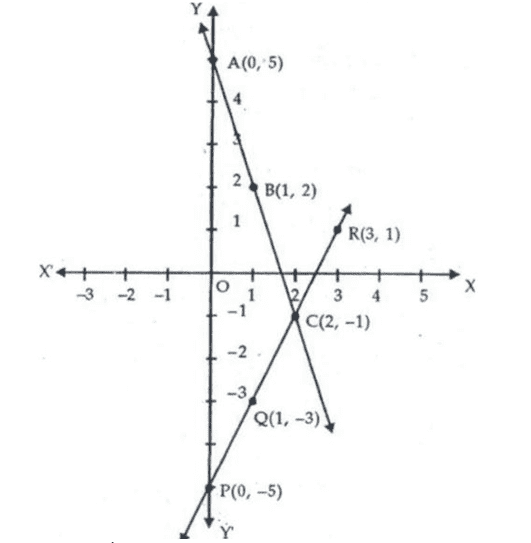The lines (1) and (2) intersect y-axis at (0, 5) and (0, -5) respectively.

Question 5: On a graph paper, draw a horizontal line X’OX and a vertical line YoY’ as the x-axis and the y-axis respectively.

3x + 5y = 15,

x – y = 5

Solution:

Given equations are 3x + 5y = 15 and x – y = 5

Graph of 3x + 5y = 15:

3x + 5y = 15 $\Rightarrow y = \frac{-3x + 15}{5}$

Putting x = 0, we get y = 3

Putting x = 5, we get y = 0

Putting x = -5, we get y = 6

Hence, the table is,

x05-5y306

Plot the points A(0, 3), B(5, 0) and C(-5, 6) on the graph paper. Join AB and AC to get the graph line BC. Extend it both ways.

Thus, line BC is the graph of 3x + 5y = 15.

Graph of x – y = 5:

x – y = 5 $\Rightarrow y = x – 5$

Putting x = 0, we get y = -5

Putting x = 5, we get y = 0

Putting x = 2, we get y = -3

Hence, the table is,

x052y-50-3

Now, on the same graph paper as above plot the points P(0, -5) and Q(2, -3). Join PQ and QB to get PB. Thus, the line PB is the graph of x – y = 5.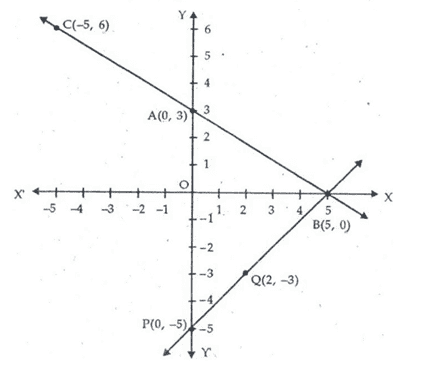It is clear from the graph that the given system of equations is consistent.

The lines 3x + 5y = 15 and x – y = 5 meet the y-axis at A(0, 3) and P(0, -5) respectively.

Question 6: On a graph paper, draw a horizontal line X’OX and a vertical line YoY’ as the x-axis and the y-axis respectively.

x + 2y = 5,

2x – 3y = -4

Solution:

Given equations are x + 2y = 5 and 2x – 3y = -4

Graph of x + 2y = 5:

x + 2y = 5 $\Rightarrow y = \frac{5 – x}{2}$

Putting x = 1, we get y = 2

Putting x = 3, we get y = 1

Putting x = 5, we get y = 0

Hence, the table is,

x135y210

Plot the points A(1, 2), B(3, 1) and C(5, 0) on the graph paper. Join AB and BC to get the graph line AC. Extend it both ways.

Thus, line AC is the graph of x + 2y = 5.

Graph of 2x – 3y = -4:

2x – 3y = -4 $\Rightarrow y = \frac{2x + 4}{3}$

Putting x = 1, we get y = 2

Putting x = -2, we get y = 0

Putting x = 4, we get y = 4

Hence, the table is,

x1-24y204

Now, on the same graph paper as above plot the points P(4, 4) and Q(-2, 0). The point A(1, 2) has already been plotted. Join PA and QA to get PQ. the line PQ is the graph of the equation 2x – 3y = -4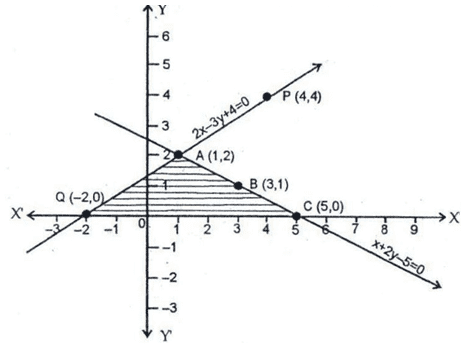The two graph lines intersect at point A(1, 2)

Therefore, x = 1, y = 2 is the solution of the given systems of equations. The region bounded by these lines and x-axis has been shaded.

On extending the graph lines on both sides, we find that these graph lines intersect x-axis at points Q(-2, 0) and C(5, 0)

Question 7: On a graph paper, draw a horizontal line X’OX and a vertical line YoY’ as the x-axis and the y-axis respectively.

4x – 5y + 16 = 0, 2x + y – 6 =0

Solution:

Given equations are 4x – 5y + 16 = 0 and 2x + y – 6 =0

Graph of 4x – 5y + 16 = 0:

4x – 5y + 16 = 0 $\Rightarrow y = \frac{4x + 16}{5}$

Putting x = 1, we get y = 4

Putting x = -4, we get y = 0

Putting x = 6, we get y = 8

Hence, the table is,

x1-46y408

Plot the points A(1, 4), B(-4, 0) and C(6, 8) on the graph paper. Join AB and AC to get the graph line BC. Extend it both ways.

Thus, line AC is the graph of 4x – 5y + 16 = 0.

Graph of 2x + y – 6 =0:

2x + y – 6 =0 $\Rightarrow y = -2x + 6$

Putting x = 1, we get y = 4

Putting x = 3, we get y = 0

Putting x = 2, we get y = 2

Hence, the table is,

x132y402

Now, on the same graph paper as above plot the points P(3, 0) and Q(2, 2). The point A(1, 4) has already been plotted. Join PQ and QA to get PA. the line PQ is the graph of the equation 2x + y – 6 =0. The two graph lines intersect at A(1, 4)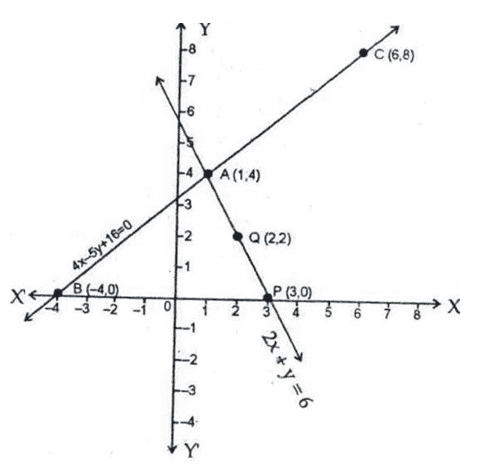Therefore, x = 1, y = 4 is the solution of the given systems of equations. Clearly, the given equations are represented by the graph lines BC and PA respectively.

The vertices of $\Delta BAP$ formed by these lines and x-axis are B(-4, 0), A(1, 4) and P(3, 0).

### Key Features of RS Aggarwal Class 10 Solutions Chapter 3 – Linear Equations In Two Variables Ex 3A (3.1)

• Solving the questions will boost up your confidence while attempting the final question paper.
• It provides all the important questions from each topic from the exam point of view.
• Practicing exercise questions of RS Aggarwal Class 10 Solutions Chapter 3 – Linear Equations in Two Variables will speed up your accuracy level.
• All the solutions are solved in a proper step-wise manner for your better understanding.

#### Practise This Question

Assertion 1: Wings of a bat and a bird are analogous organs.

Assertion 2: Forelimbs of a bat and a bird are homologous organs.

Reason : Wings of a bat and a bird do not share a common origin.